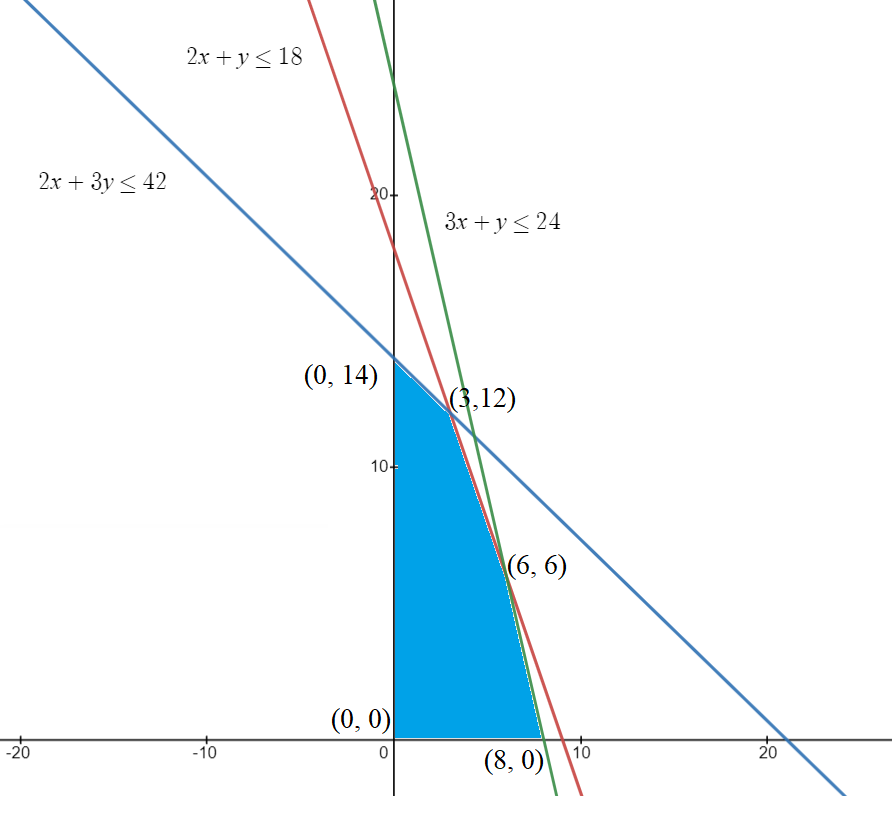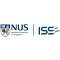Linear Programming Problem (LPP)

# Linear Programming Problem (LPP)

clickHere
Updated on Jun 12, 2023 17:14 IST

Linear Programming problem or LPP is a method to find the optimum solution of set of parameters that are represented in linear form. In this article, we will discuss all about Linear programming problem such as types of lpp, methods to solve and finally its application.

True optimization is the revolutionary contribution of modern research to decision processes.

~George Dantzig

Linear Programming Problem, or LPP, is a mathematical method to determine the best possible outcome for a given set of parameters represented in linear relationships. This article will briefly discuss LPP, its definitions, basic terminologies, methods to solve the Linear Programming Problem, and its applications.

Must Check: Top Math Courses for Data Science

## What is Linear Programming Problem or LPP?

Definition

Linear Programming problem is a technique that helps to find the optimal solution for a given problem modeled as a set of linear relationships. It is a type of optimization problem that determines the feasible region (a region that contains all the possible solutions of an LPP) and optimizes the solution to get the maximum or minimum function value.

In simple terms, a linear programming problem is a set of linear equations that are used to find the value of variables to optimize the objective functions.

### Basic Terminologies

• Decision Variable: These are quantities to be determined.
• Objective Function: The function (value) that need to optimized.
• Constraints: Represents on decision variables how to use the available resource (amount of time, number of people, etc.)
• Feasible Solution: Set all the possible solutions that satisfy the given constants.
• Optimal Solution: It is the best possible solution among all the possible feasible solutions.

### Characteristics of LPP

• Decision variables will decide the output.
• The objective function should be specified quantitatively.
• Constraints (limitations) should be expressed in mathematical form.
• Relationships between two or more variables should be linear.
• The values of the variables should always be non-negative or zero.
• There should always be finite and infinite inputs and output numbers.

For a Linear Programming problem, Decision Variable, Objective Function, and Constraint function should always be linear functions.

## Formulation of LPP

• Identify the decision variable.
• Write the Objective Function
• Write the constraints
• State the non-negative restrictions

### Standard Form of LPP

Any Linear Programming Problem can be written as:where

• aij and bj’s are constants,
• xi’s are variables (decision variables).

Note:

• All the constraints are written in equalities (rather than inequalities).
• If constraints are not in the standard form, they can be converted to the standard form by adding or subtracting the slack or surplus variable, respectively.

Then, add a slack variable (xn+1) in the above equation, i.e.,

and if we have,

Then, subtract a surplus variable ( xn+1 ) from the above equation, i.e.,

• All the decision variables are required to be positive.

## Types of Linear Programming Problems

Following are the types of Linear Programming Problems:

• Manufacturing Problem
• Diet Problem
• Transportation Problem
• Optimal Assignment ProblemTransportation Problem: Definition, Formulation, and Types
Transportation problems are used to find the minimum cost of transportation of goods from m source to n destination. In this article we will learn transportation problem, formulation, types and...read moreDifference Between Transportation Problem and Assignment Problem
In this article, we will learn what is transportation problem, what is assignment problem, and the difference between them with the help of examples.

## Methods to solve Linear Programming Problems

There are different methods to solve any Linear Programming Problem.

• Graphical Method
• Simplex Method
• North West Corner Method
• Least Square Methods

For now, we will discuss only Graphical method to solve LPP

### Graphical Method

Steps for Graphical Method:

• Formulate the LPP.
• Construct the graph and plot all the constraint lines.
• Determine the valid side of each constraint line.
• Identify the feasible solution region.
• Find the optimum points.
• Calculate the coordinates of optimum points.
• Evaluate the objective function at the optimum point.

Example: Solve the given linear programming problem by graphical method.

Max Z = f(x, y) = 3x + 2y

Constraints: 2x + y <= 18

2x + 3y <= 42

3x + y <= 24

x >= 0, y >= 0

Now, let’s plot the graph of the given constraints and find the feasible region.The above-shaded region (blue color) is the feasible region for the given lpp.
Now, we will find the value of the objective function at the corner points of the feasible region.

From the above table, we got that the objective function obtains its maximum value at (3, 12).

Hence, the maximum value of the objective function f(x,y) = 3x + 2y = 33.

## Application of Linear Programming Problem

Linear Programming Problems has their application across different domains like manufacturing/ transportation/ sports/ share market/ engineering/ energy industry/ machine learning, etc. But here, we will discuss some of its real-life applications.

• Let ‘n’ number of tasks and ‘m’ number of people completing the task. Now, assign the task so that overall productivity is optimum. We will get the optimum solution by setting the minimum cost, time is taken by each person or maximum profit. This type of problem is a type of Optimal Assignment Problem.
• Manufacturing Industries: Industries use lpp models to maximize efficiency with minimum operational cost.
• Transport Company: Company like ola, uber, and Rapido uses LPP to optimize delivery routes. The objective of these companies is to reduce time and operational costs and increase revenue.
• Machine Learning: Supervised learning works on the fundamental of linear programming.

## Conclusion

In this article, we have discussed all about linear programming problem (lpp), methods to solve, its application.

Hope you will like the article.

Keep Learning!!

Keep Sharing!!

## FAQs

What is LPP?

Linear Programming problem (LPP) is a technique that helps to find the optimal solution for a given problem modeled as a set of linear relationships. It is a type of optimization problem that determines the feasible region (a region that contains all the possible solutions of an LPP) and optimizes the solution to get the maximum or minimum function value.

What are the different applications of LPP?

Applications of LPP: 1. Resource Allocation 2. Production Planning 3. Transportation and Logistics 4. Financial Planning 5. Energy Management 6. Marketing and Advertising

clickHere

Vikram has a Postgraduate degree in Applied Mathematics, with a keen interest in Data Science and Machine Learning. He has experience of 2+ years in content creation in Mathematics, Statistics, Data Science, and Mac... Read Full Bio

## Trending Data Science CoursesAI For Everyone
Coursera4.5Machine Learning
Coursera4.6Data Science with Python
UDEMY4.2Become a Data Science Professional with IIT Certification in Advanced ProgrammingBecome a Data Science Professional with IIT Certif...
Guvi5.0Starts 15 Dec## Top Picks & New Arrivals